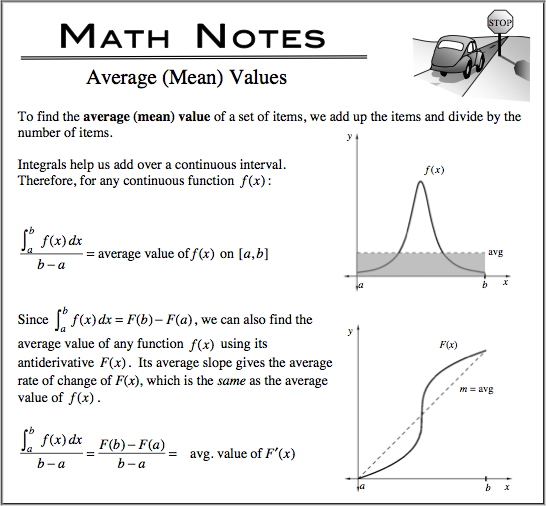### Home > CALC > Chapter 8 > Lesson 8.3.1 > Problem8-103

8-103.
1. Multiple Choice: A particle has a velocity of v(t) = t + sin t on the interval 0 ≤ t π. The average velocity of the particle is: Homework Help ✎

1. 1

2.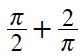3.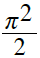4.5.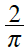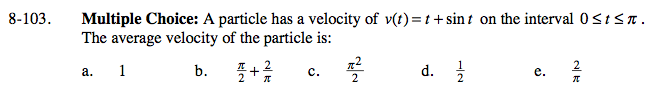You are given a velocity function and you want to find the average velocity... which variation of Average (Mean) Values will you apply? Will you compute the area under the curve and then divide? or Will you find the average rate of change: slope of the secant?